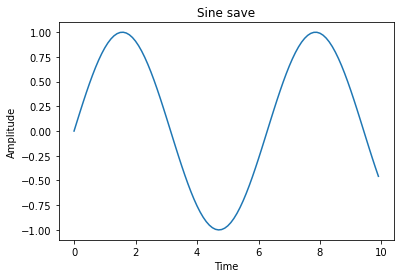## Introducing: Jupyter Notebooks

Jupyter notebooks are a great way to create tutorials and experiments. They allow for text explanations and python code and the output of the python code to be shown in the same document. I have just enabled Jupyter notebook support on this site so expect lots of interesting notebooks in the future!

### Visualisations

``````import numpy as np
import matplotlib.pyplot as plt

time = np.arange(0, 10, 0.1)
amplitude = np.sin(time)

plt.title("Sine save")
plt.plot(time, amplitude)

plt.xlabel("Time")
plt.ylabel("Amplitude")

plt.show()``````